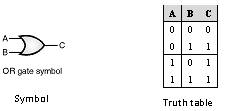Home > VHDL > Logic Gates > OR Gate

Figure below shows the symbol and the truth table of the OR gate. The OR gate can be implemented using the data flow modelling or the behavioural modelling. In data flow modelling of OR gate the operator OR is used to logically OR the inputs A and B. In behavioural modelling the behaviour of the gate is explained with the help of sequential statements.library IEEE;

use IEEE.std_logic_1164.all;

entity OR2 is

port (A,B : in std_logic;

C : out std_logic);

end OR2;

architecture data_flow of OR2 is

begin

C <= A OR B;

end data_flow;

library IEEE;

use IEEE.std_logic_1164.all;

entity OR2 is

port (A,B : in std_logic;

C : out std_logic);

end OR2;

architecture behav of OR2 is

begin

process (A,B)

begin

if (A='0' and B = '0') then

C <= '0';

elsif (A='0' and B = '1') then

C <= '1';

elsif (A='1' and B = '0') then

C <= '1';

else C <= '1';

end if;

end process;

end behav;